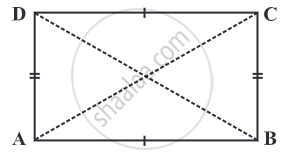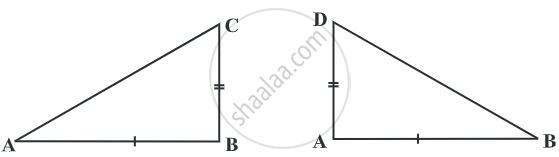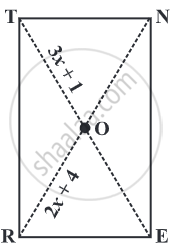# Properties of Rectangle - Property: The Diagonals of a Rectangle Are of Equal Length.

## Theorem

### The diagonals of a rectangle are of equal length.Given: ABCD is a rectangle. The diagonals are AC and BD bisect each other at a point O.

To prove: AC = BD

Proof:
ABCD is a rectangle.
BC = AD                         ...........(Opposite sides are equal and parallel)(1)
m∠A = m∠ B = 90°.       ...........(Each of the angles is a right angle and opposite angles of a rectangle are equal.)(2)

Then looking at triangles ABC and ABD separately.We have,

In ∆ ABC and ∆ABD,
AB = AB                       ......(Common side)
m∠A = m∠ B = 90°.    ......(From 2)

by SAS congruency criterion,
∆ ABC ≅ ∆ ABD          .....(lies between two parallel lines)

Thus, AC = BD           ......(C.S.C.T.)

Hence Proved.

## Example

RENT is a rectangle. Its diagonals meet at O. Find x, if OR = 2x + 4 and OT = 3x + 1.bar"OT"  "is half of the diagonal"  bar"TE".

bar"OR"  "is half of the diagonal"  bar"RN".

by the property of Diagonals of a Rectangle Are of Equal Length.

So, their halves are also equal.
Therefore,
3x + 1 = 2x + 4
3x - 2x = 4 - 1
x = 3.
If you would like to contribute notes or other learning material, please submit them using the button below.

### Shaalaa.com

To Prove that the two Diagonals of a Rectangle Are of Equal Length. [00:03:47]
S
0%